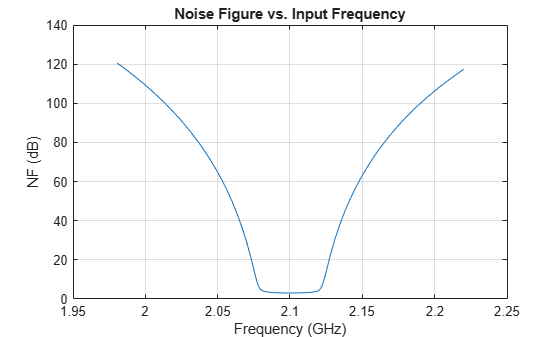Frequency Sweep in RF Budget Analysis

This example shows how to sweep through frequency-dependent properties of the elements in an RF Budget Analysis.

Use the nport and amplifier objects to specify the 2-port RF elements in the design and build an RF budget element by cascading the elements together into an RF system with rfbudget.

Building Elements of RF Budget Cascade

Build and parameterize each of the 2-port RF elements.

f1 = nport('RFBudget_RF.s2p','RFBandpassFilter');
a1 = amplifier('Name','RFAmplifier', ...
'Gain',11.53, ...
'NF',1.53, ...
'OIP3',35);

Use rfbudget object to cascade the elements with input frequency 2.1 GHz, input power -30 dBm, and input bandwidth 45 MHz. This example cascades a filter and an amplifier.

b = rfbudget('Elements',[f1 a1], ...
'InputFrequency',2.1e9, ...
'AvailableInputPower',-30, ...
'SignalBandwidth',45e6);

Read frequency-dependent Noise Figure (NF) values of the amplifier from the data-sheet. A similar approach can be followed if the Output third-order intercept (OIP3) or Gain is frequency-dependent.

% Inputs from the data-sheet
freq_datasheet = [1.98;1.99;2.0;2.01;2.02;2.03;2.04;2.05;2.06;2.07;2.08;....
2.09;2.10].*1e9;

NF_datasheet = [1.0000;1.0442;1.0883;1.1325;1.1767;1.2208;1.2650;1.3092;...
1.3533;1.3975;1.4417;1.4858;1.5300];

% Interpolate the amplifier NF data based on existing filter frequencies
Freq = f1.NetworkData.Frequencies;
RFAmplifier_NF = interp1(freq_datasheet,NF_datasheet,Freq);

Plot RF Budget Results Versus Input Frequency

Loop over the desired frequencies, by setting NF of the RF Amplifier element in the rfbudget object.

TotalNF = zeros(size(Freq));
for i = 1:numel(Freq)
b.InputFrequency = Freq(i);

% Adjust frequency-dependent NF of the RF Amplifier
elems(2).NF = RFAmplifier_NF(i);

% Compute NF of the cascade
TotalNF(i) = b.NF(end);
end
plot(Freq/1e9,TotalNF)
grid on;
xlabel('Frequency (GHz)')
ylabel('NF (dB)')
title('Noise Figure vs. Input Frequency')